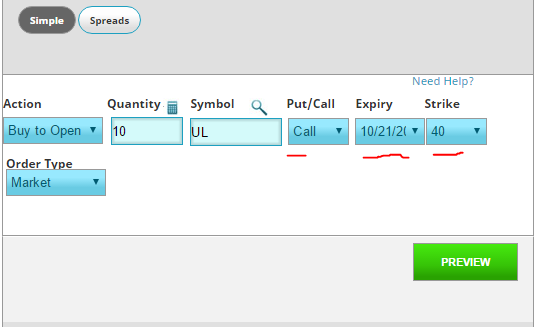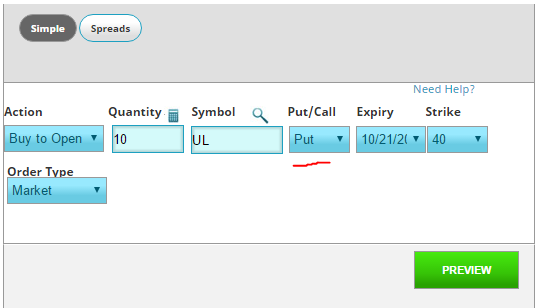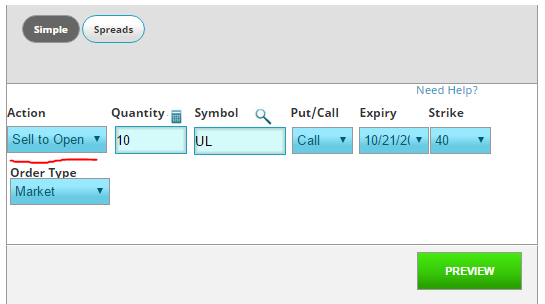# Creating Option StraddlesA straddle is an investment strategy that involves the purchase or sale of an option allowing the investor to profit regardless of the direction of movement of the underlying asset, usually a stock.

There are two straddle strategies, a long straddle and a short straddle.

# How to create a Long Straddle position

A long straddle involves a long position, where an investor purchases both a call option and a put option, both with identical strike prices and identical expiration dates.

A profit is made if the underlying asset moves significantly from the strike price in either direction.

An investor would use a straddle strategy when the market is volatile, and the investor is unsure of the direction of a stock, but certain that a large price movement will occur in either direction.

## Example of a Long Straddle Strategy

We will use an example of a Long Straddle on Unilever stock ( UL). In this example, Unilever is trading at \$40.00. They have an earnings release coming up, and we expect this release to cause the price to move up or down, but we don’t know in which direction.

To make a “Straddle”, we would place two trades: a “Call” and a “Put”, with the same strike price and expiration.Note that to make the straddle, we are placing two separate “Simple” option trades.

## Making a Profit

For simplicity, assume that each option contract costs \$5. Thus we:

• Buy 40 put contracts costing \$200.
• Buy 40 call contracts for \$200.

The trade has cost us a total of \$400 to enter both positions. Even if things go horribly wrong, we cannot lose more than this \$400.

If UL is trading at \$50 at expiration, the 40 put contracts expire worthless, but the JUL 40 call contracts expire in the money with an intrinsic value of \$900.

The investor’s profit (or loss) is calculated by subtracting the intrinsic value from the initial investment = \$900 – \$400 = \$300.

Suppose that on expiration, UL has not moved at all, so the stock price is the same as our strike price.

Both the call and put positions expire worthless and have no intrinsic value.

The investor’s profit (loss) is = \$0-\$400 = (\$400).

The investor realizes a total loss when the stock closes on expiration date at exactly the strike price therefore having no intrinsic value.

# How to create a Short Straddle position

A short straddle strategy involves simultaneously selling a put and a call of the same underlying security, having the same strike price and same expiration date.

Since the investor is selling options, their risk is theoretically unlimited, but there is a ceiling to the profits.

Unlike a long straddle, an investor can expect a profit when there is little volatility, so you would create a short straddle if you expect the stock to stay constant until the expiration of your contracts. An investor gains when the stock closes on expiration date at the target price.

## Example of a Short Straddle strategy

Our example will be identical to the one above, but the key difference is that we “Sell to Open” instead of “Buy to Open”:For simplicity, assume that each option contract costs \$5. Thus we:

• Sell 40 put contracts costing \$200.
• Sell 40 call contracts for \$200.

Thus our total revenue from the sales is \$400. This is our Maximum Profit.

If UL has strong buying activity for several weeks and climbs to \$50 (25% gain), the put contracts will expire worthless, but the 40 call contracts expires in the money, with an intrinsic value of \$400.

Our profit (loss) is calculated as the difference between the initial net credit and the intrinsic value= \$400 – \$400 = 0, so we broke even on this straddle.

Suppose the stock is still trading at \$40 on the day of expiration.

The put contracts and call contracts both have an intrinsic value of exactly 0.

Again, the investor’s profit is = \$400 – \$0 = \$400.

A maximum potential profit exists when the stock closes exactly at the strike price.

Comments are closed.